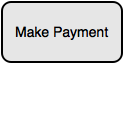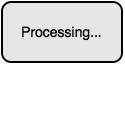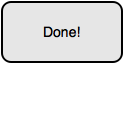# Event TimingShafaet07Cybernauts'16 National Pr...
Limits 2s, 512 MB

Bob is making a new application for Android phones. Front-end of his app has a button like this:When he press the button, a process starts in the back-end. The process needs k seconds to complete. During this k seconds the button looks like this:To check if a process is completed, Bob wrote a special checker which always runs in the background. The checker checks the progress in every d seconds starting from the moment someone pressed the button. If it sees that the process is completed, it changes the text of the button.Suppose the user pressed the button in the $p$-th second. Given the value of $p$, $k$ and $d$, can you find when the button will display “Done!”?

## Input

First line will consist $T$ ($1 \le T \le 1000$), denoting number of testcases. For each testcase, there are 3 space separated integers, $p$ ($1 \le p \le 100$), $k$ ($1 \le k \le 1000$) and $d$ ($1 \le d \le 1000$).

## Output

For each case, print the case number and the answer.

## Sample

InputOutput
1
10 14 8
Case 1: 26ImplementationuDebug

### Submit

nsucn16.42$DC4kUEarliest, Oct '16 nsucn16.108$PWmNuFastest, 0.0s